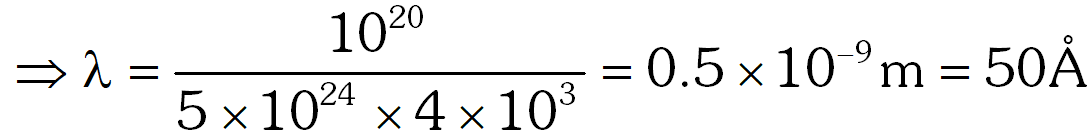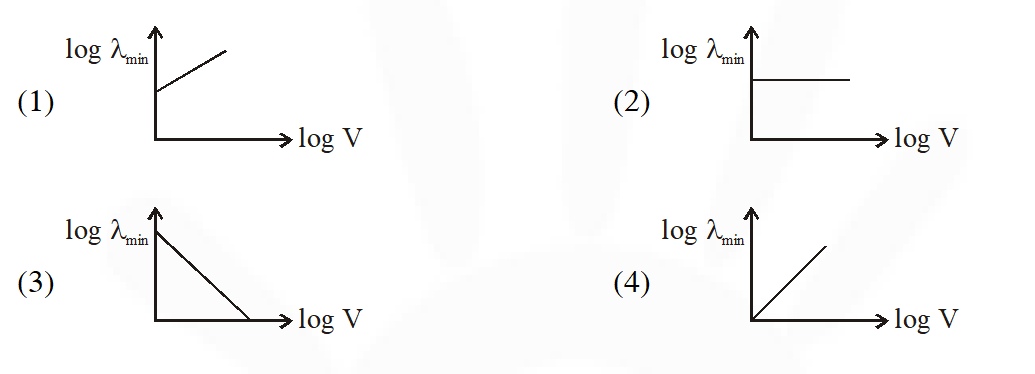Most Affordable JEE | NEET | 8,9,10 Preparation by Kota's Top IITian Doctor Faculties

# X-Rays - JEE Main Previous Year Questions with SolutionsJEE Main Previous Year Question of Physics with Solutions are available here. Practicing JEE Main Previous Year Papers Questions of Physics will help all the JEE aspirants in realizing the question pattern as well as help in analyzing their weak & strong areas. Get detailed Class 11th &12th Physics Notes to prepare for Boards as well as competitive exams like IIT JEE, NEET etc. eSaral helps the students in clearing and understanding each topic in a better way. eSaral is providing complete chapter-wise notes of Class 11th and 12th both for all subjects. Besides this, eSaral also offers NCERT Solutions, Previous year questions for JEE Main and Advance, Practice questions, Test Series for JEE Main, JEE Advanced and NEET, Important questions of Physics, Chemistry, Math, and Biology and many more. Download eSaral app for free study material and video tutorials.
Q. If a source of power 4kW produces $10^{20}$ photons/second, the radiation belongs to apart of the spectrum called :- (1) $\gamma$-rays              (2) X-rays              (3) ultraviolet rays              (4) microwaves [AIEEE - 2010]
Ans. (2) No. of photons emitting per second from a source of power $\mathrm{P}$ is $\mathrm{n}=\left(5 \times 10^{24}\right) \mathrm{P} \lambda$ $\Rightarrow$ wavelength emitting $\lambda=\frac{\mathrm{n}}{\left(5 \times 10^{24}\right) \mathrm{P}}\left[\text { or } \lambda=\frac{\mathrm{nhc}}{\mathrm{P}}\right]$And this wavlength comes in X ray region.
Q. An electron beam is accelerated by a potential difference V to hit a metallic target to produce X-rays. It produces continuous as well as characteristic X-rays.If $\lambda_{\min }$ is the smallest possible wavelength of X-ray in the spectrum, the variation of log $\lambda_{\min }$ with log V is correctly represented in :[JEE Main-2017]
Ans. (3) $\frac{\mathrm{hc}}{\lambda_{\min }}=\mathrm{eV}$ $\frac{1}{\lambda_{\text {min }}}=\frac{\mathrm{eV}}{\mathrm{hc}}$ $\ln \left(\frac{1}{\lambda_{\min }}\right)=\ell \mathrm{nV}+\ell \mathrm{n} \frac{\mathrm{e}}{\mathrm{hc}}$ $-\ell n\left(\lambda_{\min }\right)=\ell n V+\ell n \frac{\mathrm{e}}{\mathrm{hc}}$ $\ln \left(\lambda_{\min }\right)=-\ell \mathrm{n} \mathrm{V}-\ell \mathrm{n}\left(\frac{\mathrm{e}}{\mathrm{hc}}\right)$ It is a straight line with –ve slope.